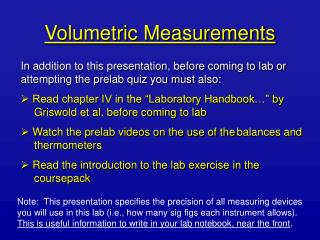# Volumetric Measurements - PowerPoint PPT PresentationDownload PresentationVolumetric Measurements

Volumetric Measurements
Download Presentation## Volumetric Measurements

- - - - - - - - - - - - - - - - - - - - - - - - - - - E N D - - - - - - - - - - - - - - - - - - - - - - - - - - -
##### Presentation Transcript

1. Volumetric Measurements • In addition to this presentation, before coming to lab or attempting the prelab quiz you must also: • Read chapter IV in the “Laboratory Handbook…” by Griswold et al. before coming to lab • Watch the prelab videos on the use of the balances and thermometers • Read the introduction to the lab exercise in the coursepack Note: This presentation specifies the precision of all measuring devices you will use in this lab (i.e., how many sig figs each instrument allows). This is useful information to write in your lab notebook, near the front.

2. What’s the point? • In this lab you will • gain experience using volumetric glassware - graduated cylinders, beakers, volumetric flasks • gain a sense of the precision and accuracy of different glassware • learn to relate significant figures in reported measurements to precision in measuring devices • practice working with significant figures and units - see Chapter 1, Brown, LeMay and Burstein

3. Different pieces of volumetric glassware have different purposes and therefore different precision and accuracy this is true of all measuring glassware; compare a measuring cup and a cake mixing bowl with volume marks The difference in precision and accuracy affect three important things: - how reproducible the measurements are - how accurate the measurements are - how many significant figures you can report Background

4. Graduated Cylinders • Use: to measure an amount of liquid to transfer • Depending on the cylinder size, they have markings for every 1 mL, 2 mL, 0.1 mL • Read the volume by finding the bottom of the meniscus - This is the curve formed by the liquid: you must be eye-level with the meniscus to read it properly • In the figure, the markings are every 2 mL, but you can read between the marks to get +/- 1 mL precision (i.e., the volume here would be reported as 100 mL, not 100.0 mL)

5. To use a graduated cylinder: • fill the cylinder to the desired mark • position yourself eye-level with the meniscus • read the volume from the bottom of the meniscus • now, you can be confident that you are transfering the desired amount of liquid

6. Beakers • Use: to transfer approximate amounts of liquids • Beakers have markings that are widely spaced. So, the volume readings are approximate • In the figure, you see two volume scales. On the right is the amount in the beaker. • Also in the figure, you can see this beaker’s precision is given as +/- 5%. • For a 250 mL beaker, that is: (0.05 x 250 mL) = 12.5 mL = +/- 10 mL • So, here we report the volume to the tens place: 200 mL, or 190 mL, but not 195 mL

7. Volumetric Flasks • Use: to contain an accurate amount of liquid • Volumetric flasks have one mark that indicates the calibrated volume at a particular temperature (e.g., 20 oC) • Virtually all are made to contain (see introduction to the lab exercise) • When the bottom of the meniscus is level with the mark, volumetric flasks have the following precisions: 5 mL: 5.00 mL 10 mL: 10.00 mL +/- 0.01 mL 25 mL: 25.00 mL +/- 0.01 mL 100 mL: 100.0 mL +/- 0.1 mL

8. Volumetric flasks are very useful for making solutions of known volume. • In this lab, we will be using them simply to investigate their accuracy and precision. • To use a volumetric flask: - fill the cylinder to the desired mark - position yourself eye-level with the meniscus - read the volume at the bottom of the meniscus - now, you can be confident that the flask contains the desired amount of liquid

9. Necessary Calculations 1) Relating volume and density Suppose you have measured the mass of H2O and have looked up its density in the CRC Handbook at a measured temperature density = mass / volume

10. Example: what is the volume if the mass of H2O is 25.032 g and its density is 0.99976 g/mL? • mass = 25.032 g • density = 0.99976 g/mL 25.032 g x 1 mL = 25.038 mL 0.99976 g

11. Necessary Calculations 2) Percent error Suppose you have measured something for which you know the true or expected value. How accurate is your measurement? Is it too small (negative error) or too big (positive error)? Percent error is one way to express this accuracy. % error = (measured - expected) x 100% expected

12. Example: you have H2O, contained in a 25-mL volumetric flask, whose volume you have calculated to be 24.752 mL. What is the percent error? % error = (measured – expected) x 100% expected A 25-mL volumetric flask contains 25.00 mL, the “expected” value. % error = (24.752 – 25.00) mL x 100% 25.00 mL Note the critical sig figs in red % error = -0.25 mL x 100% = -0.99% 25.00 mL Pay attention to sig figs in this lab!!

13. For Everyone’s Safety • your lab goggles and coat must be on whenever anyone is working in the lab • no open toed shoes are allowed in lab • move around the lab carefully to avoid bumping into other students